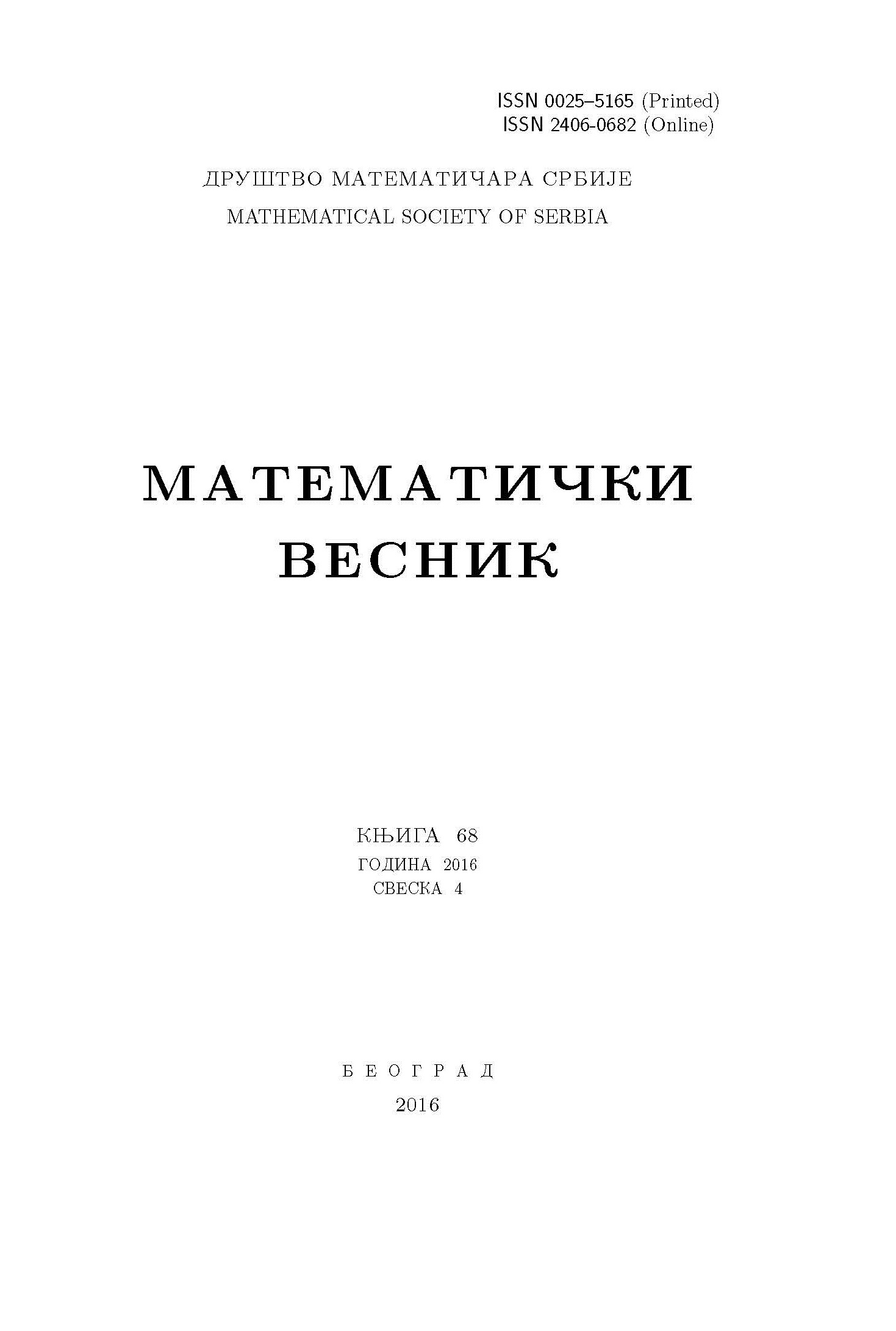﻿ Matematički Vesnik ﻿
 MATEMATIČKI VESNIK МАТЕМАТИЧКИ ВЕСНИКNEW CHARACTERIZATIONS OF FUZZY TOPOLOGY D. N. Georgiou, A. C. Megaritis, G. A. Prinos AbstractFollowing the generalization of Moore-Smith convergence of nets to fuzzy topological spaces which was given in Pu Pao-Ming, Liu Ying-Ming, \emph{Fuzzy topology I. Neighborhood structure of a fuzzy point and Moore-Smith convergence}, J. Math. Anal. Appl., \textbf{76} (1980), 571--599, a characterization theorem between fuzzy topologies and fuzzy convergence classes was introduced in Ying-Ming Liu, \emph{On fuzzy convergence classes}, Fuzzy Sets and Systems, \textbf{30} (1989), 47--51. Our goal in this paper is to provide modified versions of this characterization. Specifically, we will introduce the concept of fuzzy semi-convergence class to give an alternative characterization of fuzzy topology, in relation to the ordinary convergence of fuzzy nets, and then we will introduce the concept of fuzzy ideal convergence class to obtain analogous results, in relation to the ideal convergence of fuzzy nets.Keywords: Fuzzy set; fuzzy topology; fuzzy convergence class; fuzzy ideal convergence class. MSC: 54A20 Pages:  1$-$16

﻿